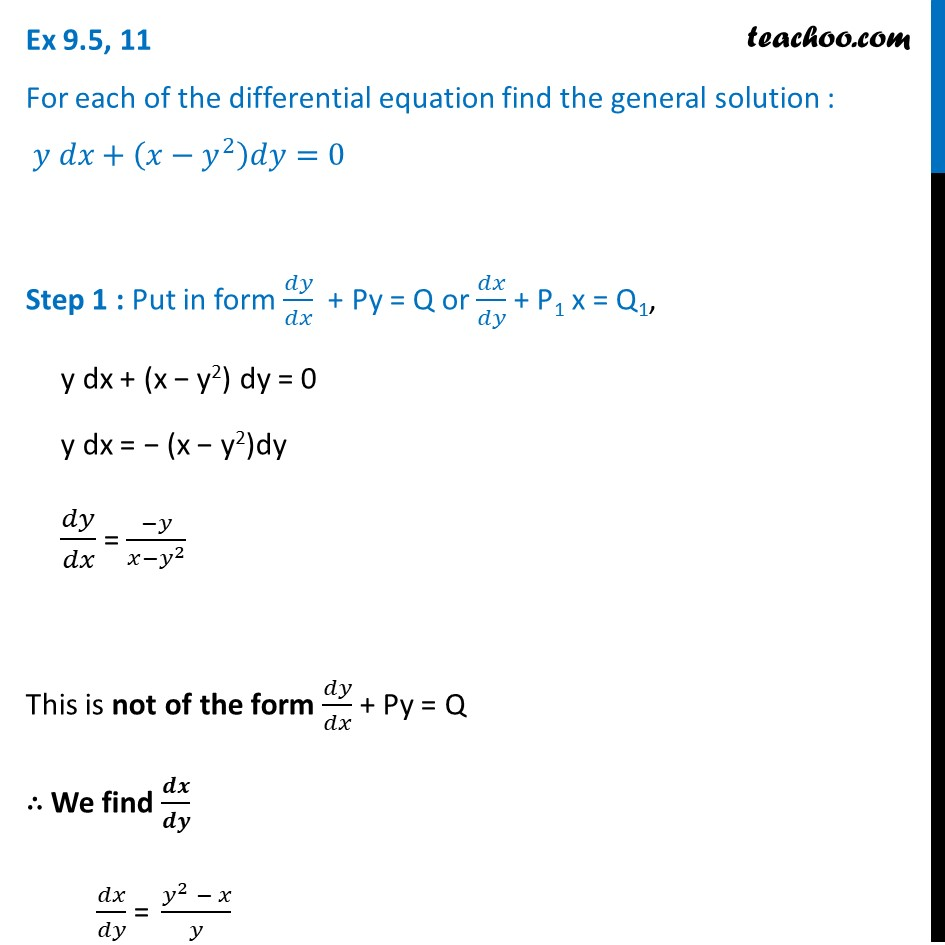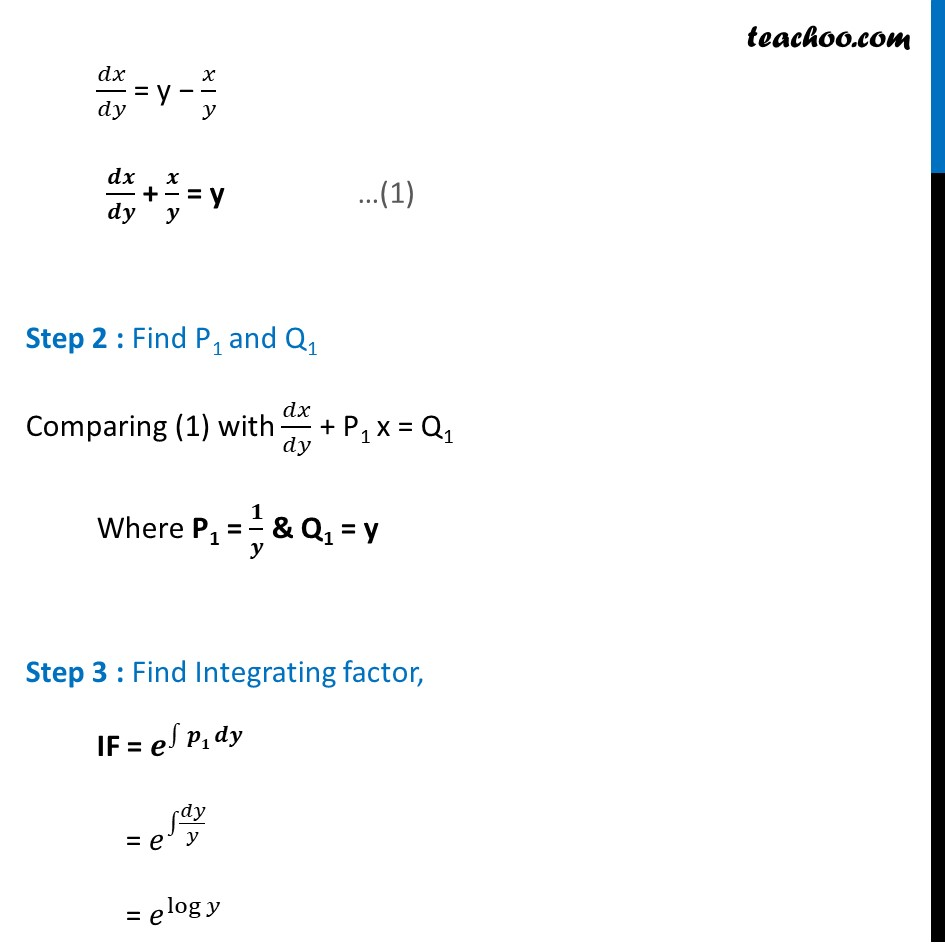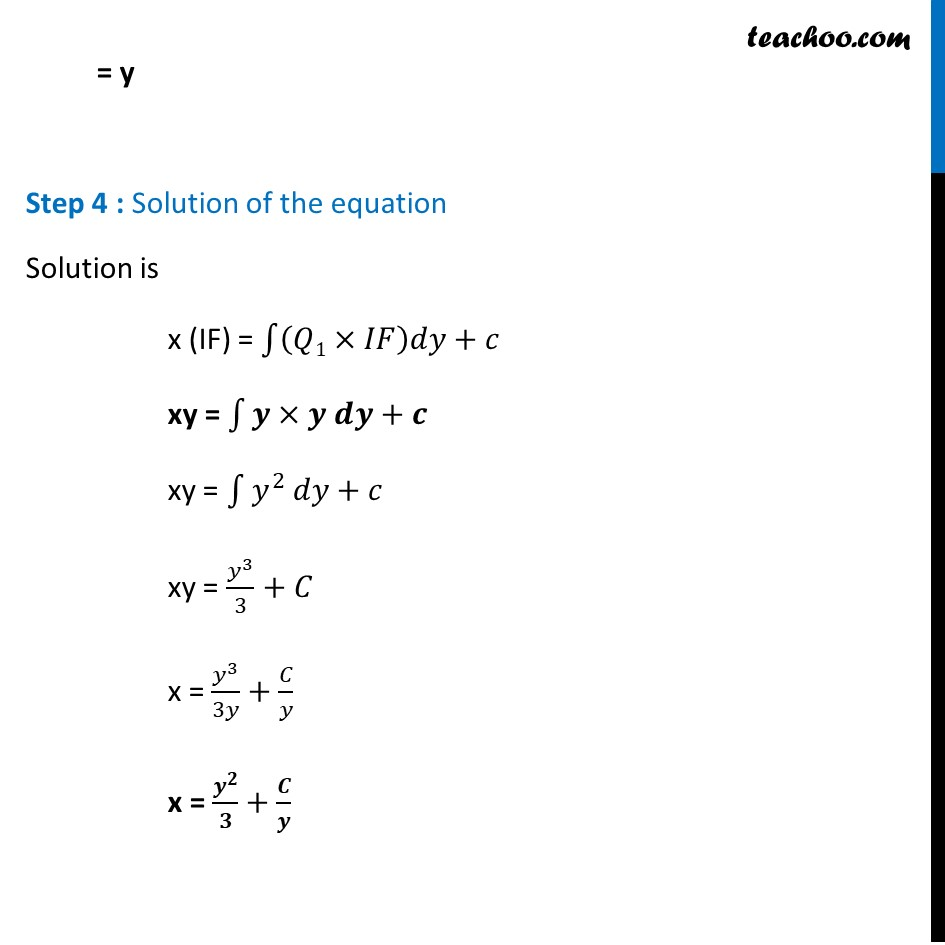Ex 9.5

Chapter 9 Class 12 Differential Equations
Serial order wiseLearn in your speed, with individual attention - Teachoo Maths 1-on-1 Class

### Transcript

Ex 9.5, 11 For each of the differential equation find the general solution : 𝑦 𝑑𝑥+(𝑥−𝑦^2 )𝑑𝑦=0 Step 1 : Put in form 𝑑𝑦/𝑑𝑥 + Py = Q or 𝑑𝑥/𝑑𝑦 + P1 x = Q1, y dx + (x − y2) dy = 0 y dx = − (x − y2)dy 𝑑𝑦/𝑑𝑥 = (−𝑦)/(𝑥−𝑦^2 ) This is not of the form 𝑑𝑦/𝑑𝑥 + Py = Q ∴ We find 𝒅𝒙/𝒅𝒚 𝑑𝑥/𝑑𝑦 = (𝑦^2 − 𝑥)/𝑦 𝑑𝑥/𝑑𝑦 = y − 𝑥/𝑦 𝒅𝒙/𝒅𝒚 + 𝒙/𝒚 = y Step 2 : Find P1 and Q1 Comparing (1) with 𝑑𝑥/𝑑𝑦 + P1 x = Q1 Where P1 = 𝟏/𝒚 & Q1 = y Step 3 : Find Integrating factor, IF = 𝒆^∫1▒〖𝒑𝟏 𝒅𝒚〗 = 𝑒^∫1▒𝑑𝑦/𝑦 = 𝑒^log⁡𝑦 = y Step 4 : Solution of the equation Solution is x (IF) = ∫1▒〖(𝑄1×𝐼𝐹)𝑑𝑦+𝑐〗 xy = ∫1▒〖𝒚×𝒚 𝒅𝒚+𝒄〗 xy = ∫1▒〖𝑦^2 𝑑𝑦+𝑐〗 xy = 𝑦^3/3+𝐶 x = 𝑦^3/3𝑦+𝐶/𝑦 x = 𝒚^𝟐/𝟑+𝑪/𝒚